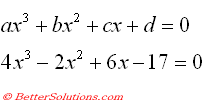# Cubic Equation

### Example

Lets consider the following cubic equation that we want to find a solution for.
One way to find a solution is to use the Solver add-in.
This add-in allows you to use a formula and specify a result in order to determine the necessary argument values.